### Set line breaks and align equations

You can control equation breaks across lines and alignment of the lines in a multiline equation, or of the items in a list or matrix. You can also align several equations with one another, even if they appear in different anchored frames.

Aligned along left side of equal signs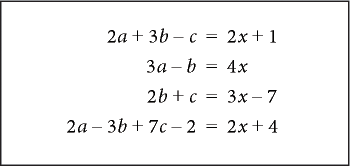You can manipulate equation objects as you do other objects. You can automatically and manually align items in a vertical list and lines in a multiline equation. Manual alignment points override auto­matic alignment.

Aligned on left side and at manual alignment point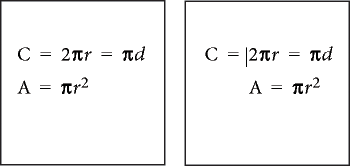You can align the items in a horizontal list along their tops, bottoms, or baselines.

Baseline-aligned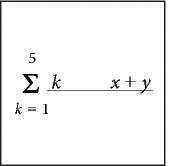You can also align the cells in each row of a matrix along their tops, bottoms, or baselines. You can align the cells in each column at the right, left, or center, or along equal signs.

Aligned along: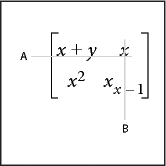A. Baselines B. Centers

#### Change equation line breaking

When an equation breaks across lines, the entire equation remains in a single anchored frame. You can set where an equation breaks across lines automatically. When you change the line-break width, the equation is reformatted to the new width.

Original equation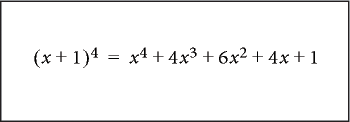Equation set to break at 1.25"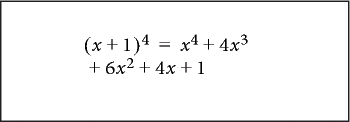You can also force a line break at a specific location in the equation. However, don’t use manual line breaks to create separate equations. Instead, insert a vertical list of expressions.

You can change line breaking in these ways:

To change the automatic line break width, click in the equation or select any part of it. Choose Graphics > Object Properties. Edit the value in the Automatic Line Break After box, and click Set. The value is preset to the width of the equation frame.

To insert a manual line break, click where you want the equation to break into two lines. On the Positioning page of the Equations pod, choose Set Manual from the Line Breaking pop-up menu. A line-break symbolappears at the insertion point when text symbols are visible.

Manual line break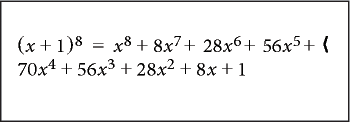To remove a manual line break, select the part of the equation that contains the line-break symbol. On the Positioning page of the Equations pod, choose Clear Manual from the Line Breaking pop-up menu.

#### Align display equations

The format of the paragraph that contains the equation controls the alignment (left, center, or right) of a display equation.

Paragraph alignment set to Center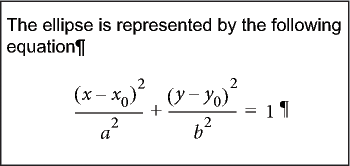1)Click in the paragraph that contains the equation (not in the equation itself).

2)Choose the alignment from the Alignment pop-up menu in the formatting bar or in the Para­graph Designer.

#### Set automatic alignment for a vertical list or multiline equation

1)Click in the equation or list.

2)On the Positioning page of the Equations pod, choose an item from the Left/Right pop-up menu. When you choose Left Of = or Right Of =, the lines are aligned along one side of an equal sign. A line with no equal sign is aligned along its left side.

##### Set a manual alignment point for a multiline equation or for an item in a vertical list

1)Do one of the following:

Click in the first line of a multiline equation where you want subsequent lines to align.

Click in a vertical list item where you want the item to align with the rest of the list.

2)On the Positioning page of the Equations pod, choose Set Manual from the Left/Right pop-up menu. A manual alignment symbolappears at the insertion point when text symbols are visible. Subsequent lines of a multiline equation are left aligned with the manual alignment point. A vertical list item aligns with other items in the list at the manual alignment point.

#### Clear a manual alignment point

1)Select the part of the equation that contains the manual alignment point.

2)On the Positioning page of Equations pod, choose Clear Manual from the Left/Right pop-up menu. You can also clear a manual alignment point by setting another.

#### Align items in a horizontal list

1)Click in the list.

2)On the Positioning page of the Equations pod, choose an item from the Up/Down pop-up menu.

#### Align cells in a matrix

1)Click in the matrix.

2)On the Positioning page of the Equations pod, choose an item from the Up/Down pop-up menu or from the Left/Right pop-up menu. The Left/Right and Up/Down commands affect the whole matrix. To align individual cells, use micropositioning.

#### Check alignment settings for a horizontal or vertical list, or for a matrix

1)Do one of the following to select the entire list or matrix:

Drag through the list or matrix.

Place the insertion point in the matrix and press the spacebar repeatedly.

2)On the Positioning Page of the Equations pod, click Position Settings.

#### Left-, center-, or right-align equations in a frame

You can align several equation objects with one another in a graphic frame, just as you align other objects. You can also align several equation objects along a manual alignment point, even when they’re in separate graphic frames.

Equations aligned at manual alignment points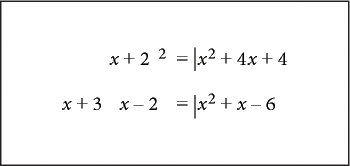FrameMaker maintains the alignment of the equation objects as you edit the equations. For example, if two equations are left aligned, the left alignment is maintained as the equations expand or shrink.

1)Select the equations to align by Control-clicking the first equation; Control+Shift-click the other equations.

Make sure that the last equation you select is the one you want to align with.

2)Choose Graphics > Align.

3)Select the left/right alignment you want, and click Align.

#### Align equations along a point

1)Set the manual alignment point in one of the equations to align.

If you don’t set a manual alignment point, FrameMaker aligns an equation on the left.

2)Click in the equation and choose Graphics > Object Properties.

3)Choose Manual from the Alignment pop-up menu and specify the location of the alignment point within the frame in the Alignment Point Offset area. The left offset is the distance from the left edge of the frame to the manual alignment point.

Specifying the top offset precisely is unnecessary. You can move the equation up or down later, just as you do any other object.

4)Click Set.

5)Repeat steps 1 through 4 for each equation you want to align. Use the same distance from the left edge for each equation, but change the top offset to position the equation vertically in the frame. If the equations you’re aligning are in different anchored frames, make sure that the left edges align with one another.

September 30, 2016